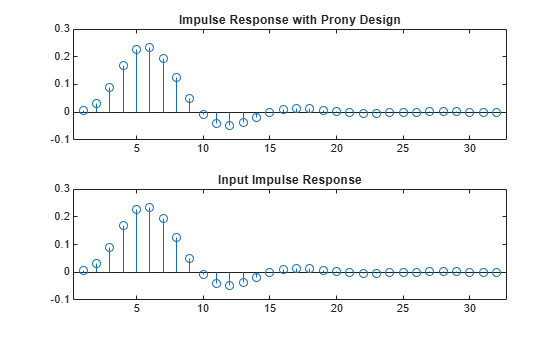# prony

Prony method for filter design

## Syntax

``[b,a] = prony(h,bord,aord)``

## Description

example

````[b,a] = prony(h,bord,aord)` returns the numerator and denominator coefficients for a causal rational transfer function with impulse response `h`, numerator order `bord`, and denominator order `aord`.```

## Examples

collapse all

Fit a 4th-order IIR model to the impulse response of a lowpass filter. Plot the original and Prony-designed impulse responses.

```d = designfilt('lowpassiir','NumeratorOrder',4,'DenominatorOrder',4, ... 'HalfPowerFrequency',0.2,'DesignMethod','butter'); h = filter(d,[1 zeros(1,31)]); bord = 4; aord = 4; [b,a] = prony(h,bord,aord); subplot(2,1,1) stem(impz(b,a,length(h))) title 'Impulse Response with Prony Design' subplot(2,1,2) stem(h) title 'Input Impulse Response'```Fit a 10th-order FIR model to the impulse response of a highpass filter. Plot the original and Prony-designed frequency responses. The responses match to high precision.

```d = designfilt('highpassfir','FilterOrder',10,'CutoffFrequency',0.8); h = filter(d,[1 zeros(1,31)]); bord = 10; aord = 0; [b,a] = prony(h,bord,aord); fvt = fvtool(b,a,d); legend(fvt,'Prony','Original')```## Input Arguments

collapse all

Impulse response, specified as a vector.

Example: `impz(fir1(20,0.5))` specifies the impulse response of a 20th-order FIR filter with normalized cutoff frequency π/2 rad/sample.

Data Types: `single` | `double`
Complex Number Support: Yes

Numerator and denominator orders, specified as positive integer scalars. If the length of `h` is less than max(`bord`,`aord`), the function pads the impulse response with zeros.

• If you want an all-pole transfer function, specify `bord` as `0`.

• If you want an all-zero transfer function, specify `aord` as `0`.

Data Types: `single` | `double`

## Output Arguments

collapse all

Transfer function coefficients, returned as vectors. `b` has length and `a` has length .

collapse all

### Transfer Function

The transfer function is the Z-transform of the impulse response h[n]:

`$H\left(z\right)=\sum _{n=-\infty }^{\infty }h\left(n\right)\text{ }\text{\hspace{0.17em}}{z}^{-n}.$`

A rational transfer function is a ratio of polynomials in z–1. This equation describes a causal rational transfer function of numerator order q and denominator order p:

`$H\left(z\right)=\frac{B\left(z\right)}{A\left(z\right)}=\frac{\sum _{k=0}^{q}b\left(k\right)\text{ }\text{\hspace{0.17em}}{z}^{-k}}{1+\sum _{l=1}^{p}a\left(l\right)\text{ }\text{\hspace{0.17em}}{z}^{-l}},$`

where a = 1.

 Parks, Thomas W., and C. Sidney Burrus. Digital Filter Design. New York, NY, USA: Wiley-Interscience, 1987.